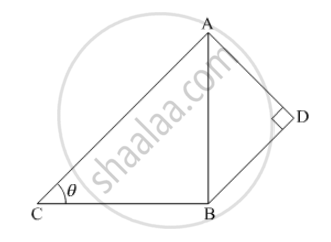Advertisement Remove all ads

# In the Following Figure. Ad = 4 Cm, Bd = 3 Cm and Cb = 12 Cm, Find the Cot θ. - Mathematics

MCQ

In the following Figure. AD = 4 cm, BD = 3 cm and CB = 12 cm, find the cot θ.#### Options

• $\frac{12}{5}$

• $\frac{5}{12}$

• $\frac{13}{12}$

• $\frac{12}{13}$

Advertisement Remove all ads

#### Solution

We have the following given data in the figure, AD=4cm, BD=3 cm, CB=12 cm

Now we will use Pythagoras theorem in, ΔABD

AB=sqrt(3^2+4^2)

= 5 cm

Therefore,

cot θ  =( CB)/(AB)

=12/5

Is there an error in this question or solution?
Advertisement Remove all ads

#### APPEARS IN

RD Sharma Class 10 Maths
Chapter 10 Trigonometric Ratios
Q 35 | Page 59
Advertisement Remove all ads
Advertisement Remove all ads
Share
Notifications

View all notifications

Forgot password?